# Vertex AI：超参数调节

## 学习内容

• 修改训练应用代码以进行自动超参数调节
• 通过 Vertex AI 界面配置和启动超参数调节作业
• 使用 Vertex AI Python SDK 配置并启动超参数调节作业

## 2. Vertex AI 简介

Vertex AI 包含许多不同的产品，可支持端到端机器学习工作流。本实验将重点介绍下列产品：培训Workbench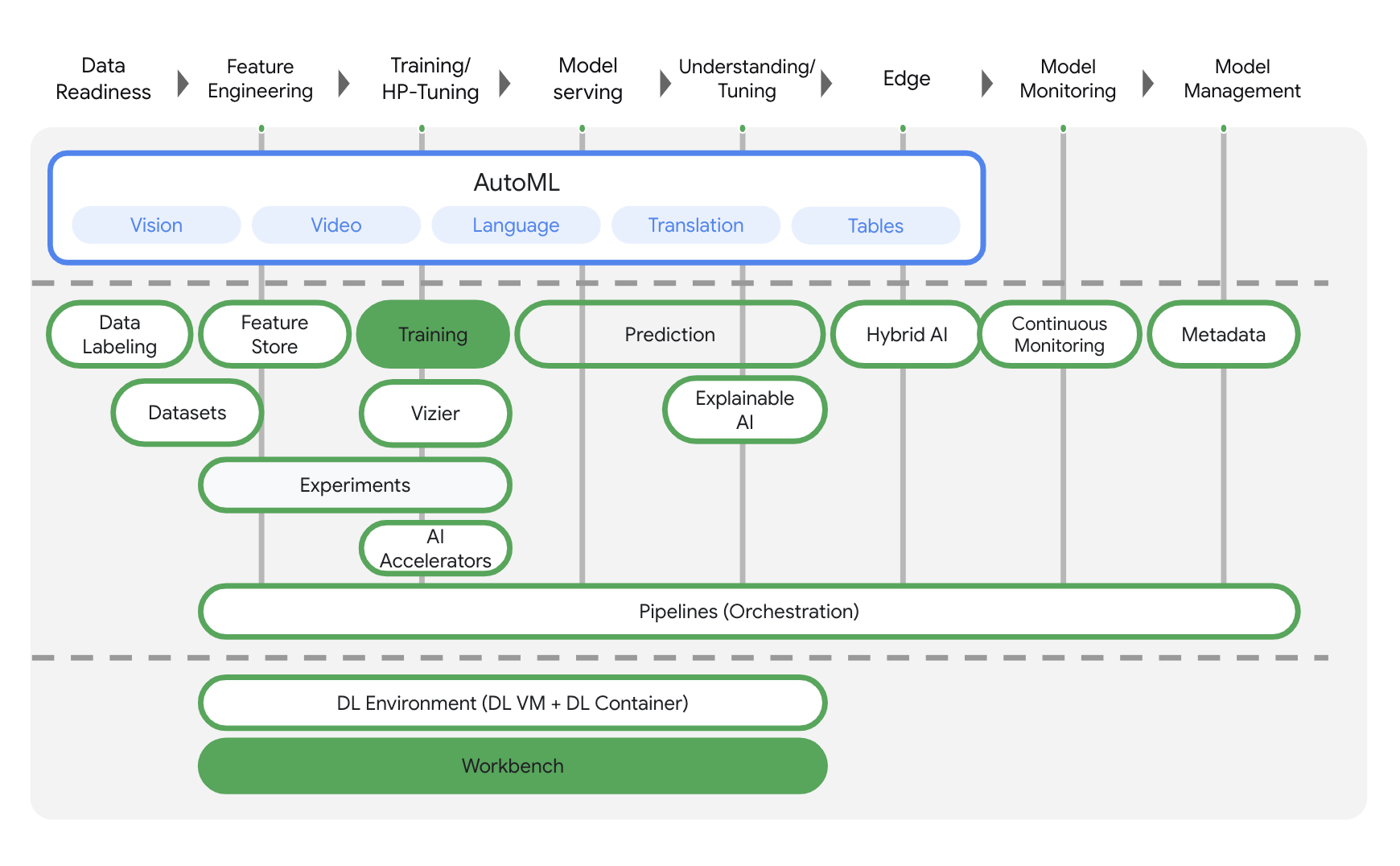## 第 3 步：启用 Vertex AI API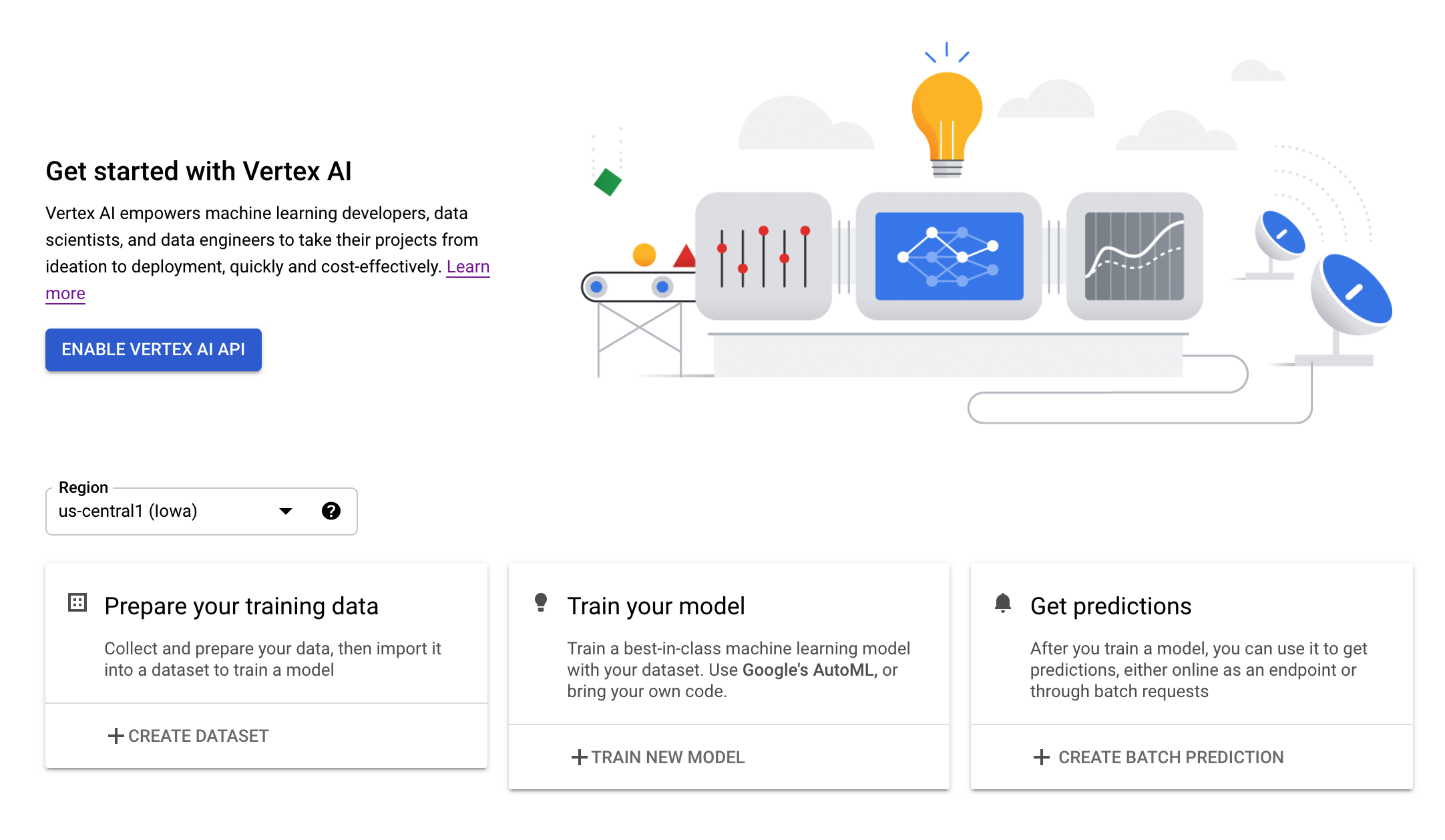## 第 4 步：创建 Vertex AI Workbench 实例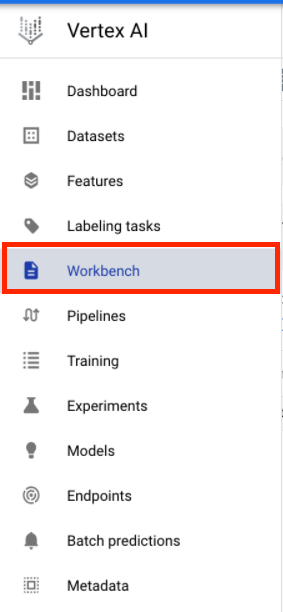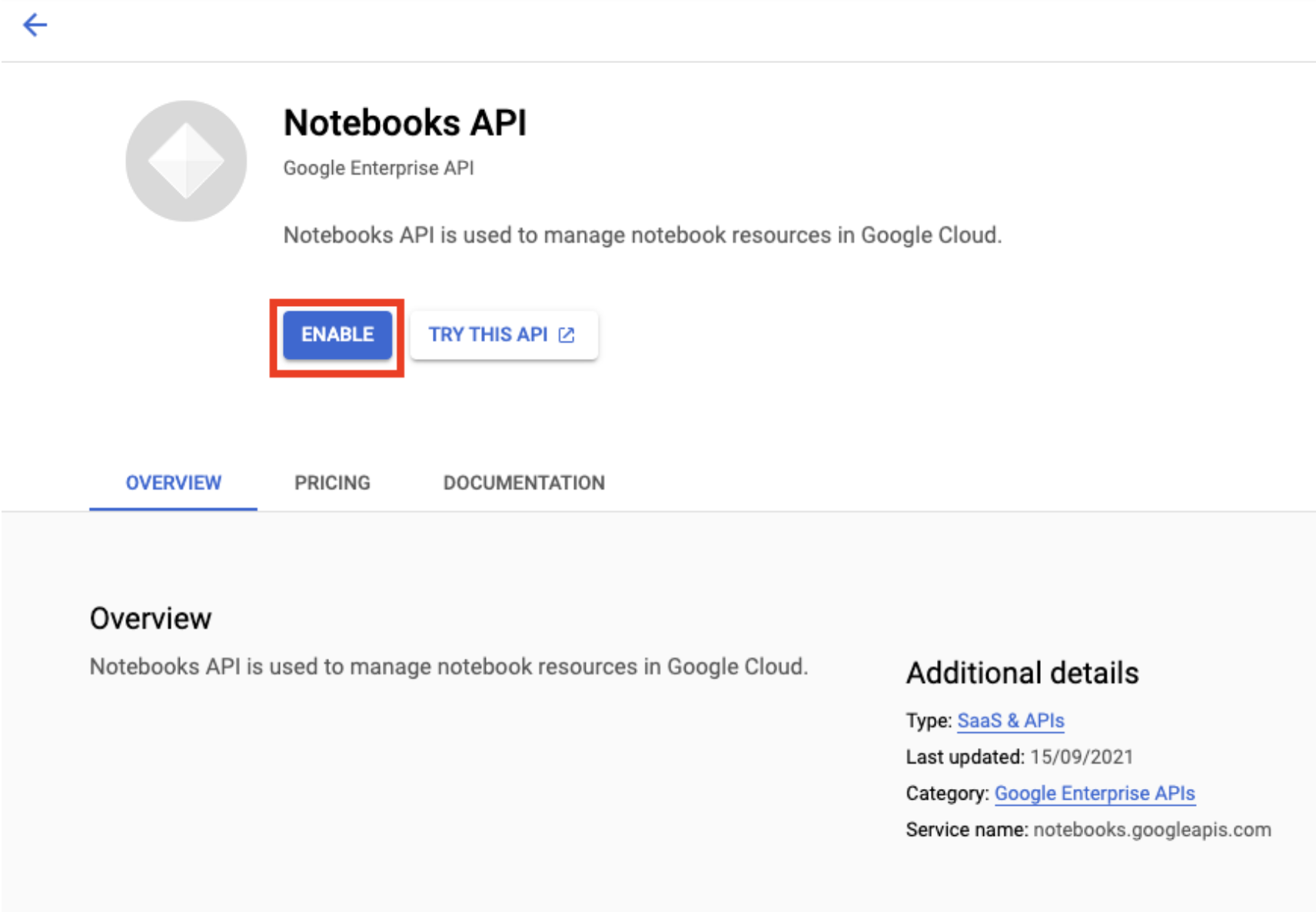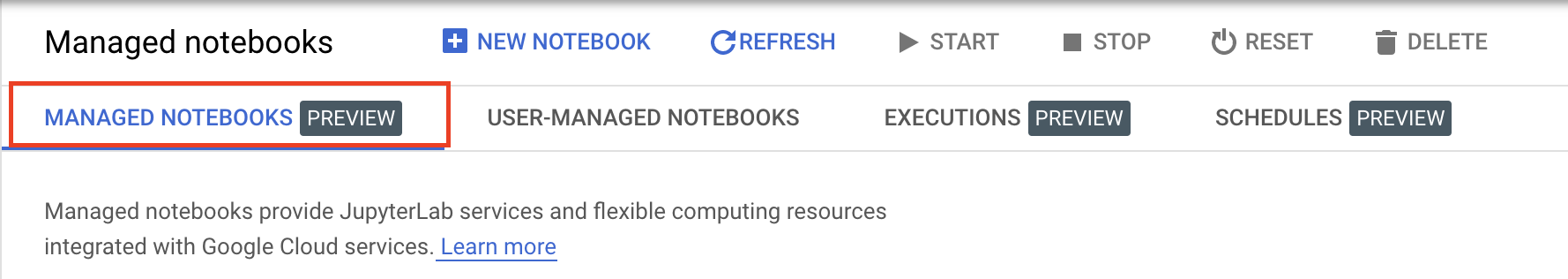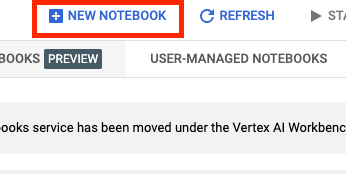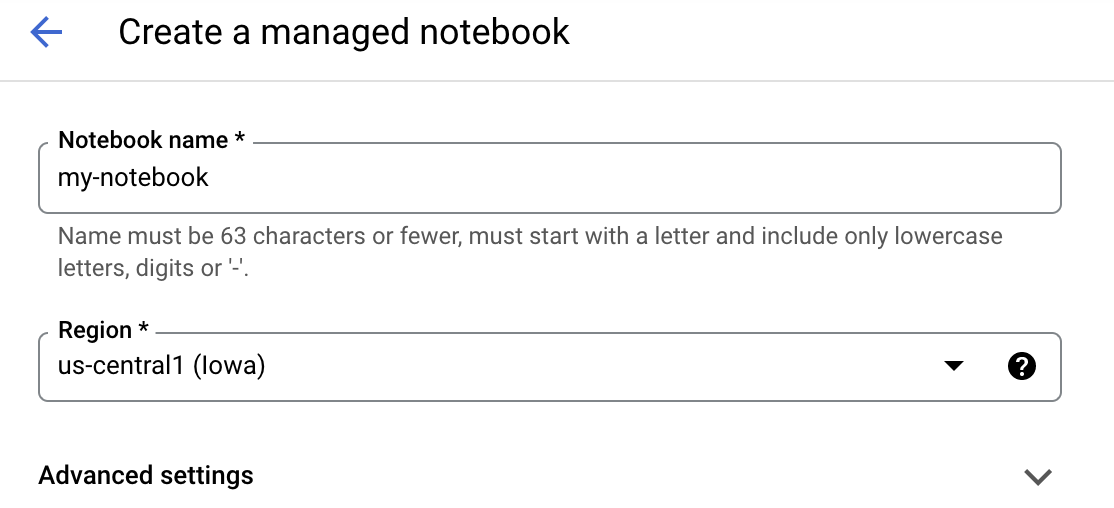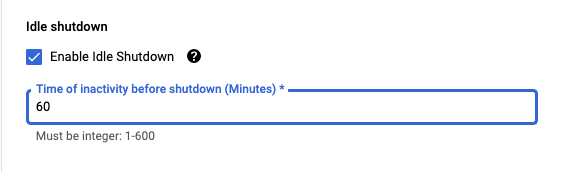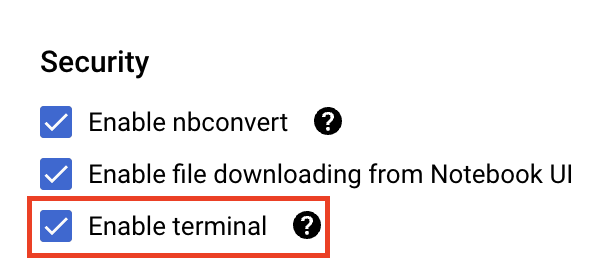## 4.将训练应用代码容器化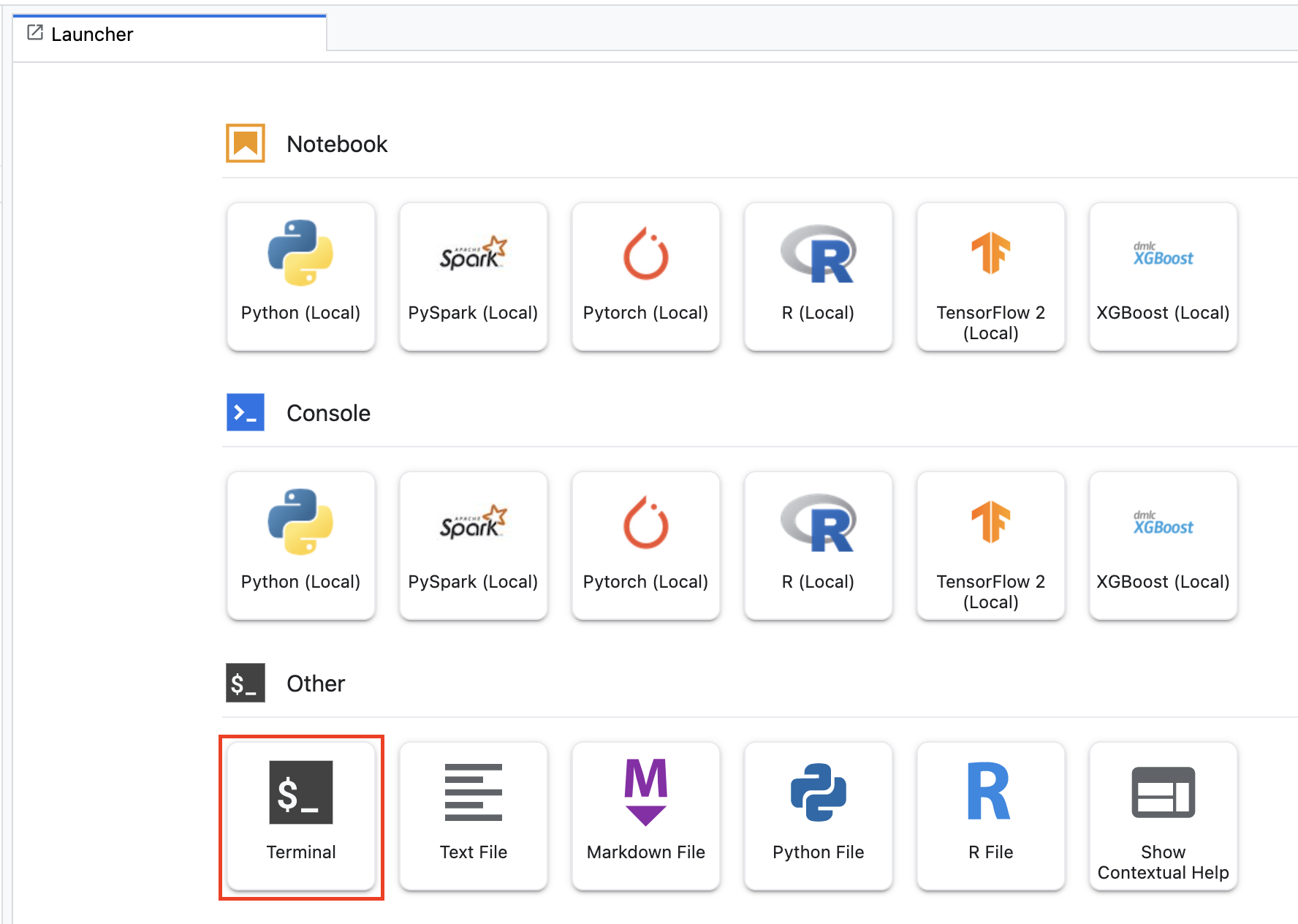``````mkdir horses_or_humans
cd horses_or_humans
``````

## 第 1 步：创建 Dockerfile

``````touch Dockerfile
``````

``````FROM gcr.io/deeplearning-platform-release/tf2-gpu.2-7

WORKDIR /

# Installs hypertune library
RUN pip install cloudml-hypertune

# Copies the trainer code to the docker image.
COPY trainer /trainer

# Sets up the entry point to invoke the trainer.
``````

## 第 2 步：添加模型训练代码

``````mkdir trainer
``````

``````+ Dockerfile
+ trainer/
``````

``````import tensorflow as tf
import tensorflow_datasets as tfds
import argparse
import hypertune

NUM_EPOCHS = 10

def get_args():
'''Parses args. Must include all hyperparameters you want to tune.'''

parser = argparse.ArgumentParser()
'--learning_rate',
required=True,
type=float,
help='learning rate')
'--momentum',
required=True,
type=float,
help='SGD momentum value')
'--num_units',
required=True,
type=int,
help='number of units in last hidden layer')
args = parser.parse_args()
return args

def preprocess_data(image, label):
'''Resizes and scales images.'''

image = tf.image.resize(image, (150,150))
return tf.cast(image, tf.float32) / 255., label

def create_dataset():
'''Loads Horses Or Humans dataset and preprocesses data.'''

data, info = tfds.load(name='horses_or_humans', as_supervised=True, with_info=True)

# Create train dataset
train_data = data['train'].map(preprocess_data)
train_data  = train_data.shuffle(1000)
train_data  = train_data.batch(64)

# Create validation dataset
validation_data = data['test'].map(preprocess_data)
validation_data  = validation_data.batch(64)

return train_data, validation_data

def create_model(num_units, learning_rate, momentum):
'''Defines and compiles model.'''

inputs = tf.keras.Input(shape=(150, 150, 3))
x = tf.keras.layers.Conv2D(16, (3, 3), activation='relu')(inputs)
x = tf.keras.layers.MaxPooling2D((2, 2))(x)
x = tf.keras.layers.Conv2D(32, (3, 3), activation='relu')(x)
x = tf.keras.layers.MaxPooling2D((2, 2))(x)
x = tf.keras.layers.Conv2D(64, (3, 3), activation='relu')(x)
x = tf.keras.layers.MaxPooling2D((2, 2))(x)
x = tf.keras.layers.Flatten()(x)
x = tf.keras.layers.Dense(num_units, activation='relu')(x)
outputs = tf.keras.layers.Dense(1, activation='sigmoid')(x)
model = tf.keras.Model(inputs, outputs)
model.compile(
loss='binary_crossentropy',
optimizer=tf.keras.optimizers.SGD(learning_rate=learning_rate, momentum=momentum),
metrics=['accuracy'])
return model

def main():
args = get_args()
train_data, validation_data = create_dataset()
model = create_model(args.num_units, args.learning_rate, args.momentum)
history = model.fit(train_data, epochs=NUM_EPOCHS, validation_data=validation_data)

# DEFINE METRIC
hp_metric = history.history['val_accuracy'][-1]

hpt = hypertune.HyperTune()
hpt.report_hyperparameter_tuning_metric(
hyperparameter_metric_tag='accuracy',
metric_value=hp_metric,
global_step=NUM_EPOCHS)

if __name__ == "__main__":
main()
``````

1. 该脚本会导入 `hypertune` 库。请注意，第 1 步中的 Dockerfile 包含对 pip 安装此库的说明。
2. `get_args()` 函数为每个要调节的超参数定义命令行参数。在本示例中，将要调整的超参数为学习速率、优化器的动量值以及模型最后一个隐藏层中的单元数，但您可以随意尝试其他参数。然后，在这些参数中传递的值将用于在代码中设置相应的超参数。
3. `main()` 函数的末尾，`hypertune` 库用于定义您要优化的指标。在 TensorFlow 中，keras `model.fit` 方法会返回 `History` 对象。`History.history` 属性用于记录连续周期中的训练损失值和指标值。如果您将验证数据传递给 `model.fit``History.history` 属性也将包含验证损失和指标值。例如，如果您使用验证数据训练了三个周期的模型，并提供了 `accuracy` 作为指标，则 `History.history` 属性将类似于以下字典。
``````{
"accuracy": [
0.7795261740684509,
0.9471358060836792,
0.9870933294296265
],
"loss": [
0.6340447664260864,
0.16712145507335663,
0.04546636343002319
],
"val_accuracy": [
0.3795261740684509,
0.4471358060836792,
0.4870933294296265
],
"val_loss": [
2.044623374938965,
4.100203514099121,
3.0728273391723633
]
``````

## 第 3 步：构建容器

``````PROJECT_ID='your-cloud-project'
``````

``````IMAGE_URI="gcr.io/\$PROJECT_ID/horse-human:hypertune"
``````

``````docker build ./ -t \$IMAGE_URI
``````

``````docker push \$IMAGE_URI
``````

## 5. 在 Vertex AI 上运行超参数调节作业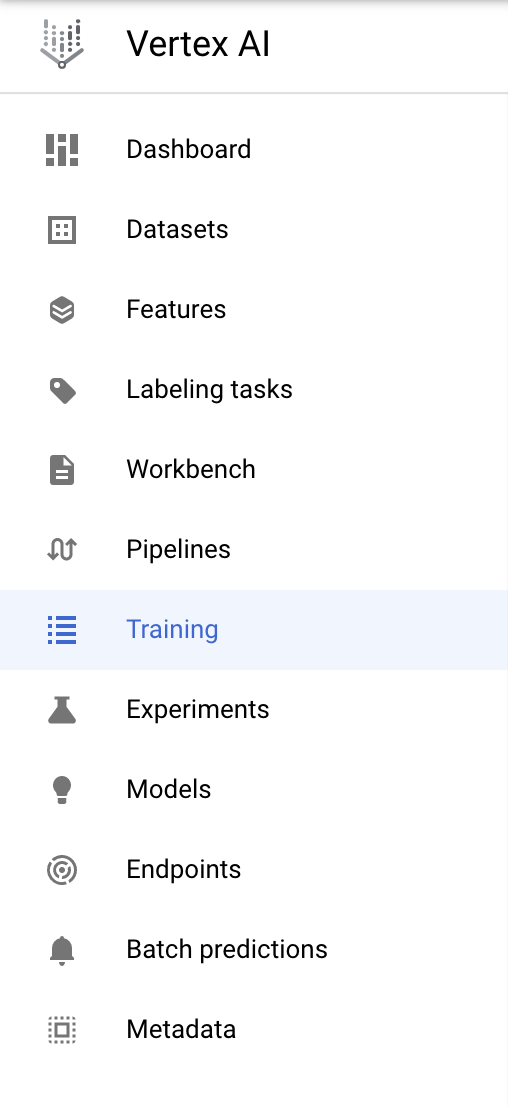## 第 1 步：配置训练作业

• 数据集下，选择无代管式数据集
• 然后选择自定义训练（高级）作为训练方法，并点击继续
• 输入 `horses-humans-hyptertune`（或您希望调用模型的任何名称）作为模型名称
• 点击继续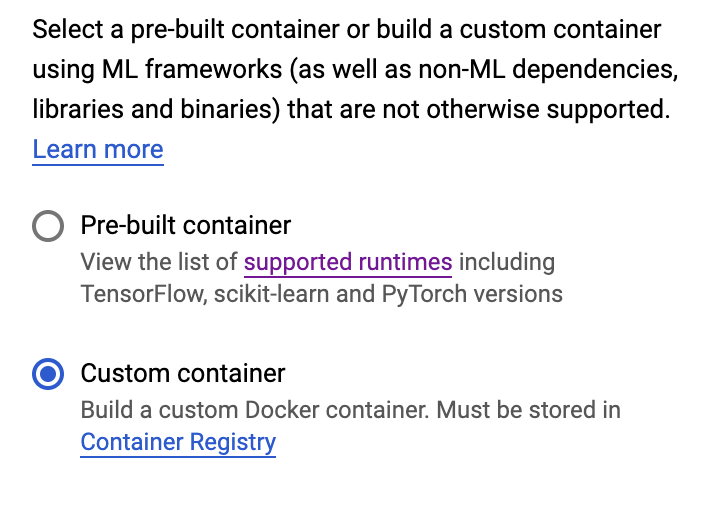## 第 2 步：配置超参数调节作业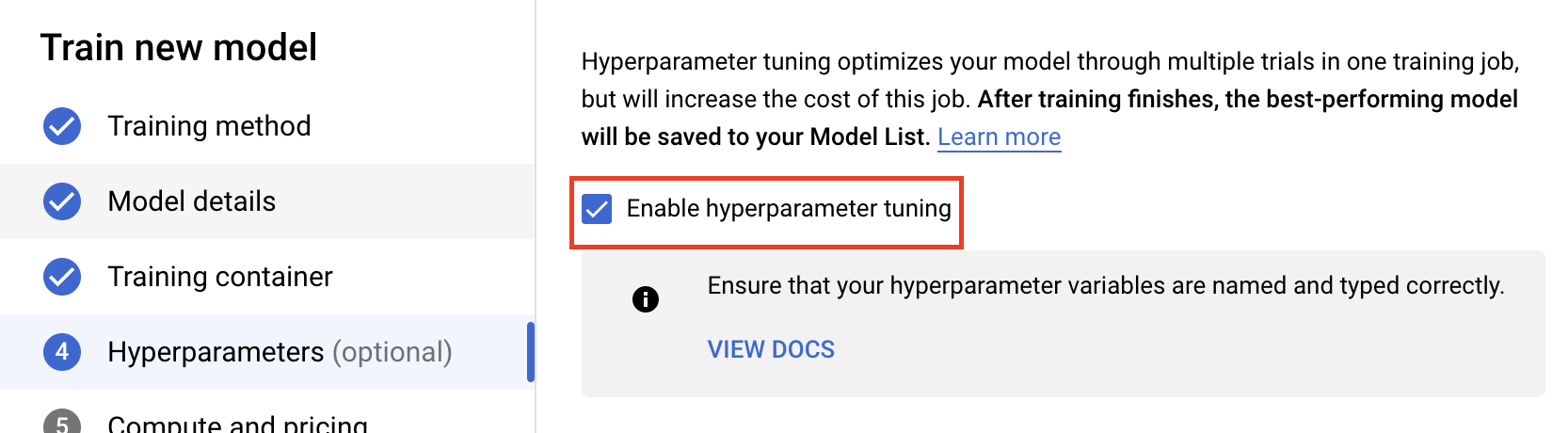### 配置超参数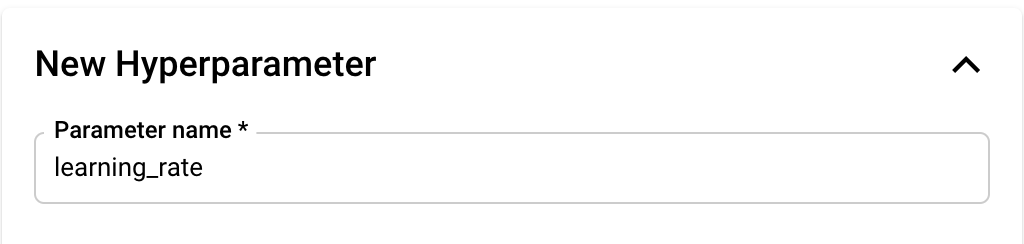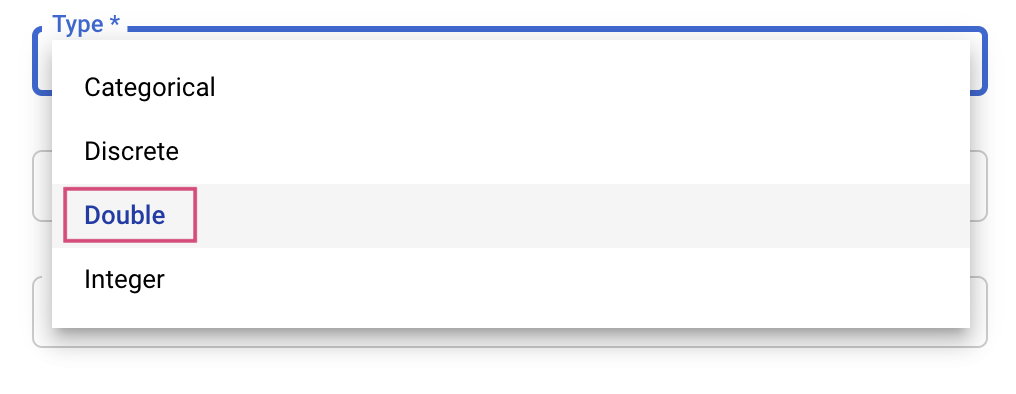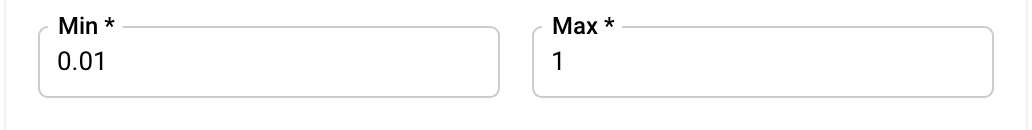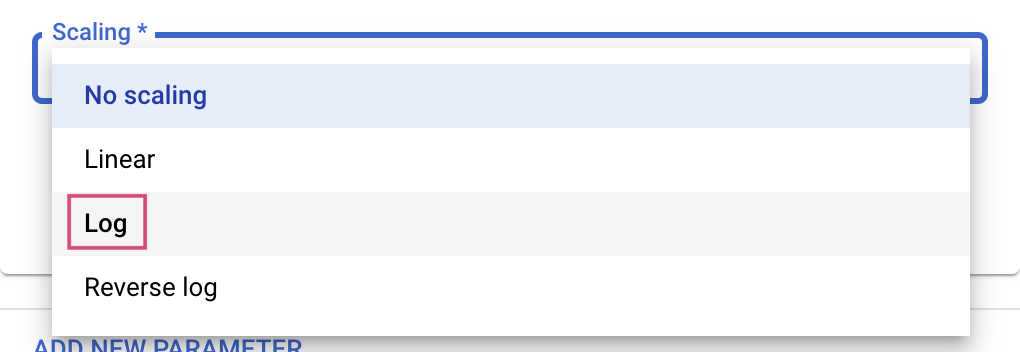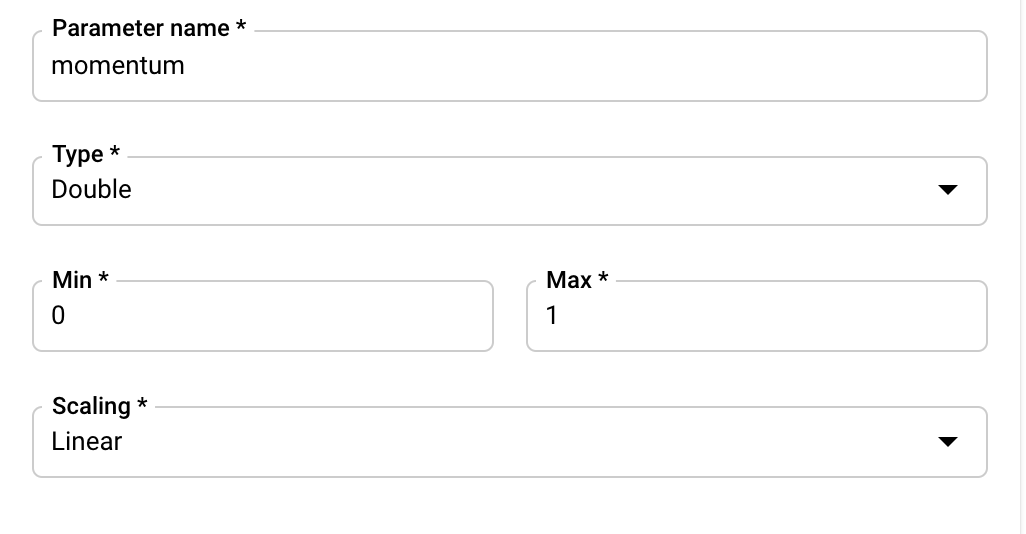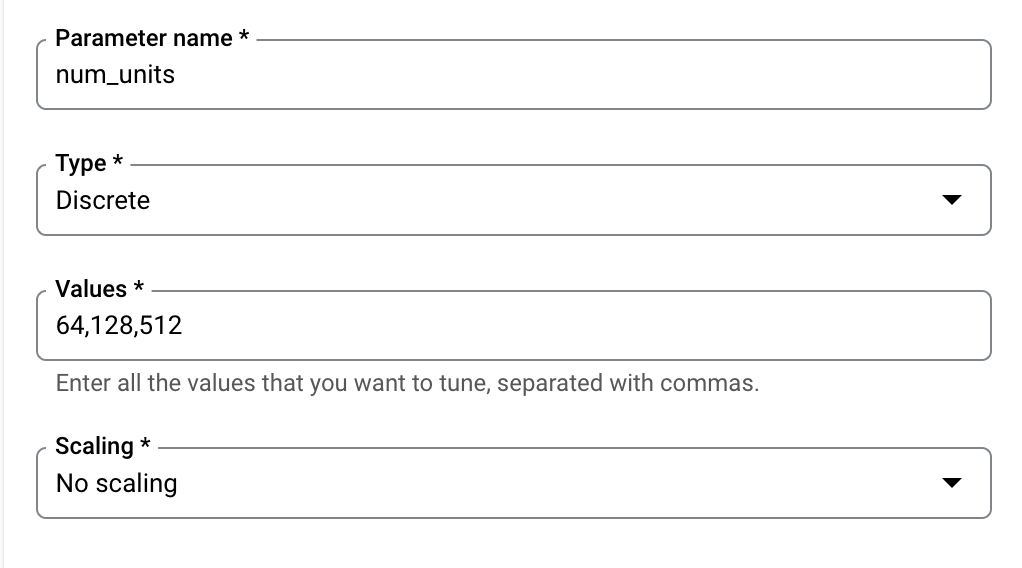### 配置指标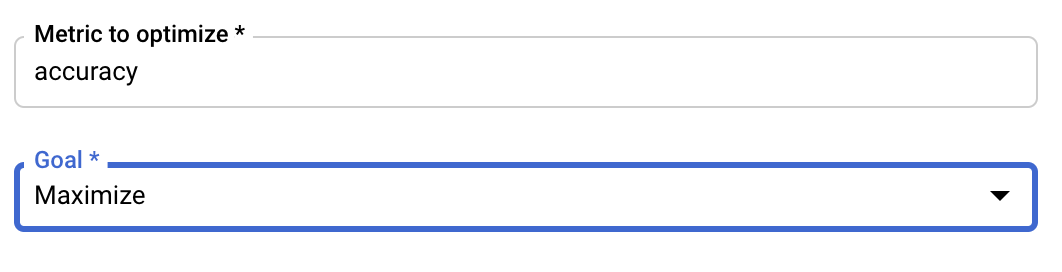Vertex AI 超参数调整服务将使用您在上一步配置的值运行训练应用的多个试验。您需要设置服务运行的试验次数的上限。实验轮次通常可以带来更好的效果，但回报率会下降，在此之后，其他轮次的试验对您想要优化的指标几乎没有或没有影响。最佳做法是先减少试验次数，并了解在超大量试验之前所选超参数的影响程度。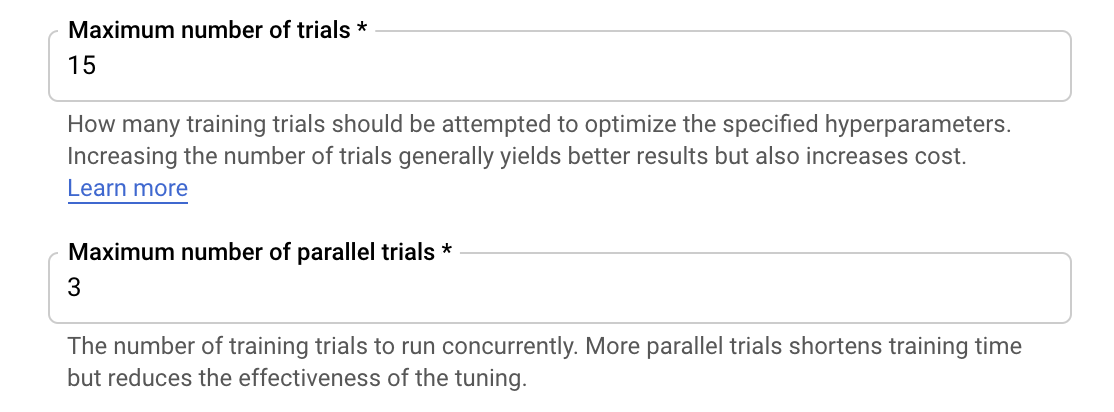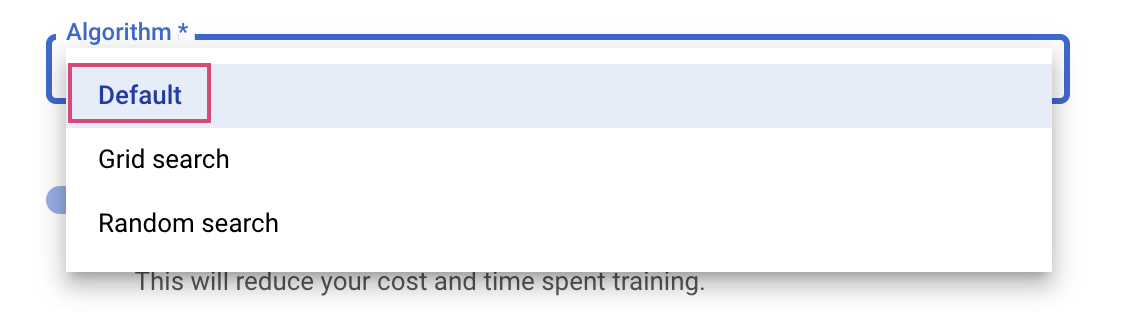## 第 3 步：配置计算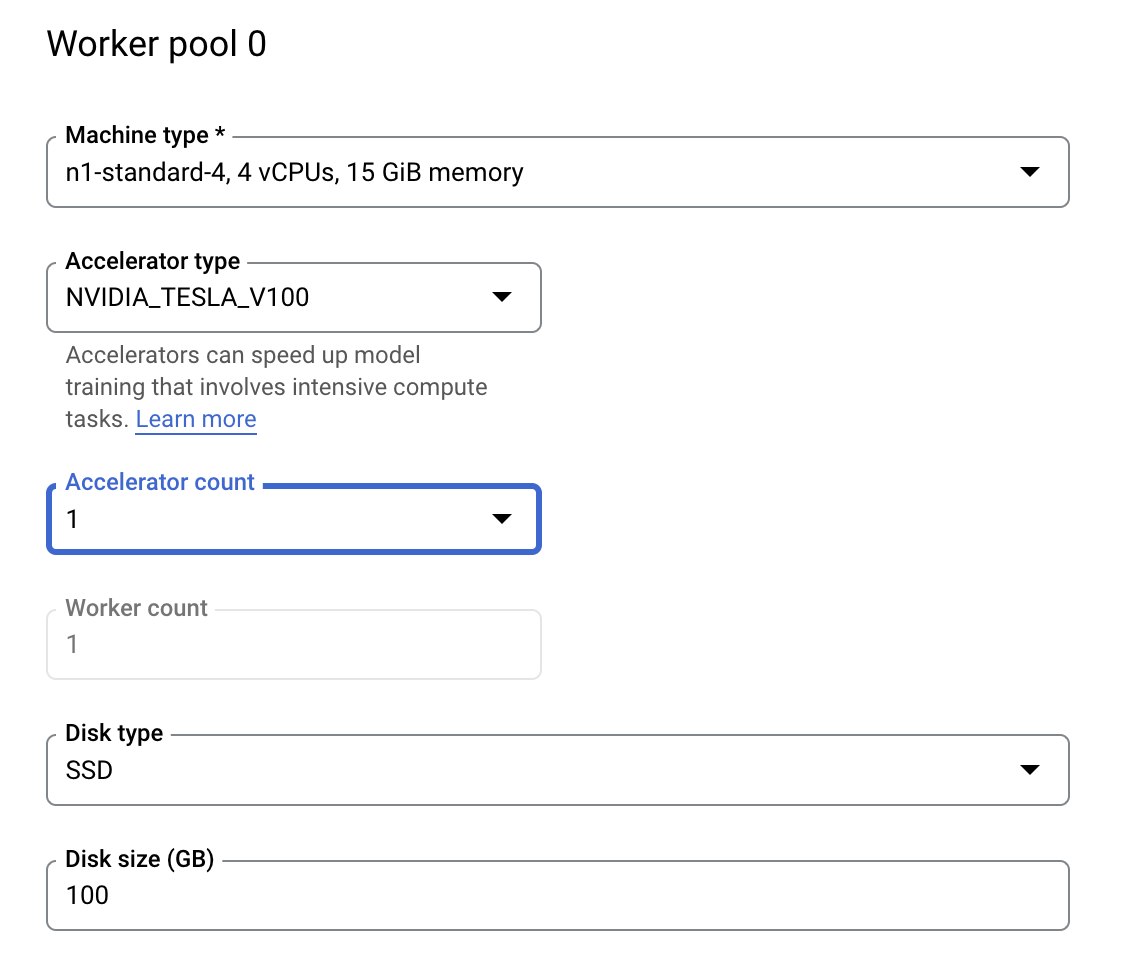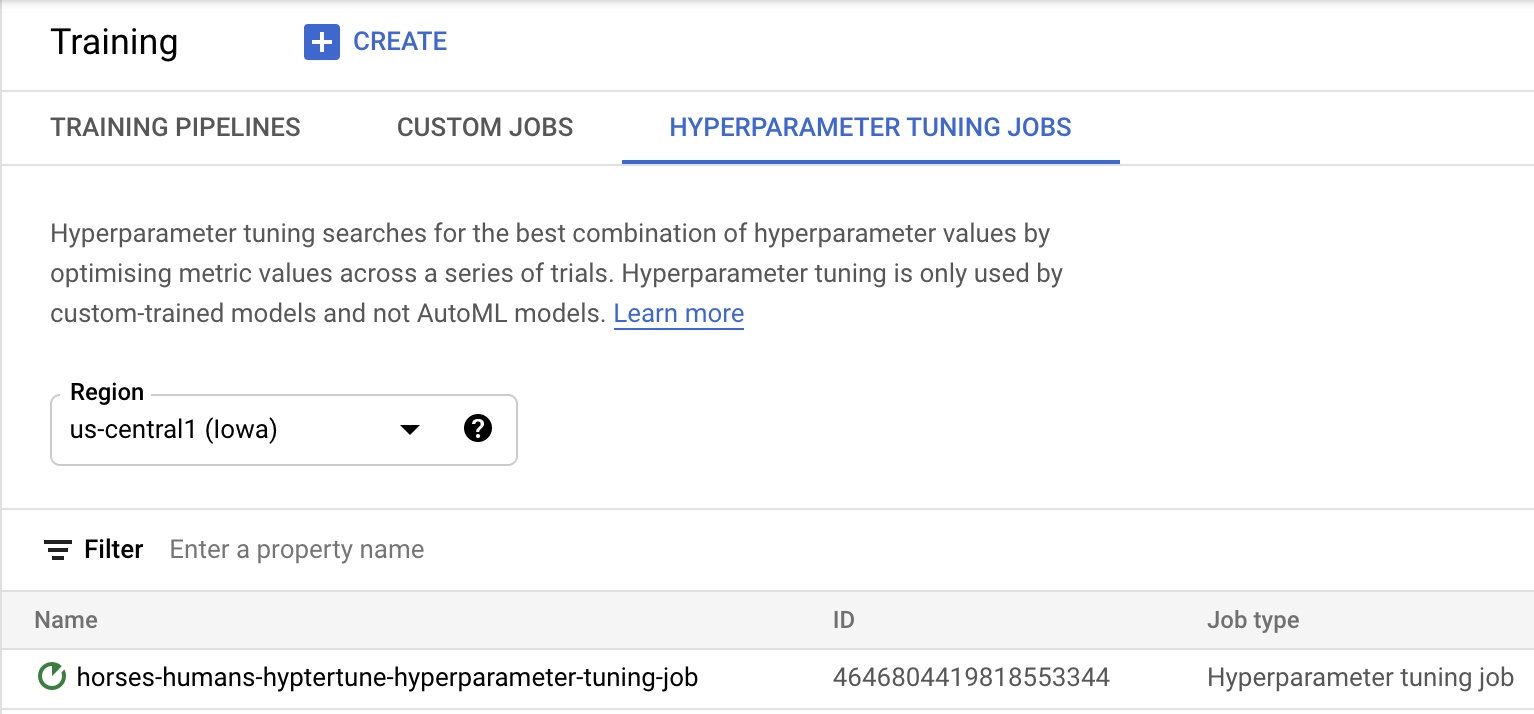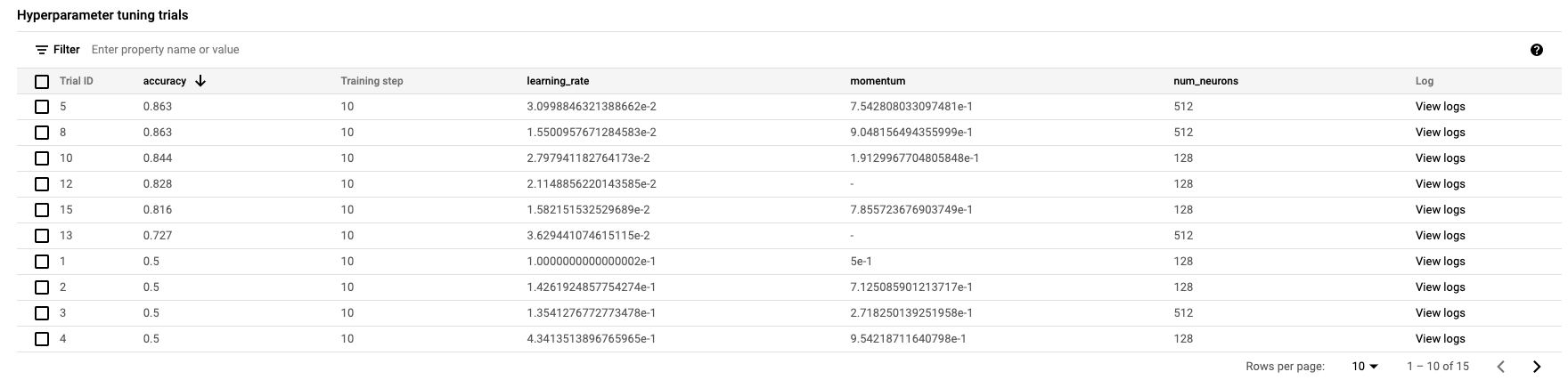🎉? 恭喜！🎉

• 启动自定义容器中提供的用于训练代码的超参数调节作业。您在本示例中使用了 TensorFlow 模型，但可以使用自定义容器训练使用任何框架构建的模型。

## 6.[可选] 使用 Vertex SDK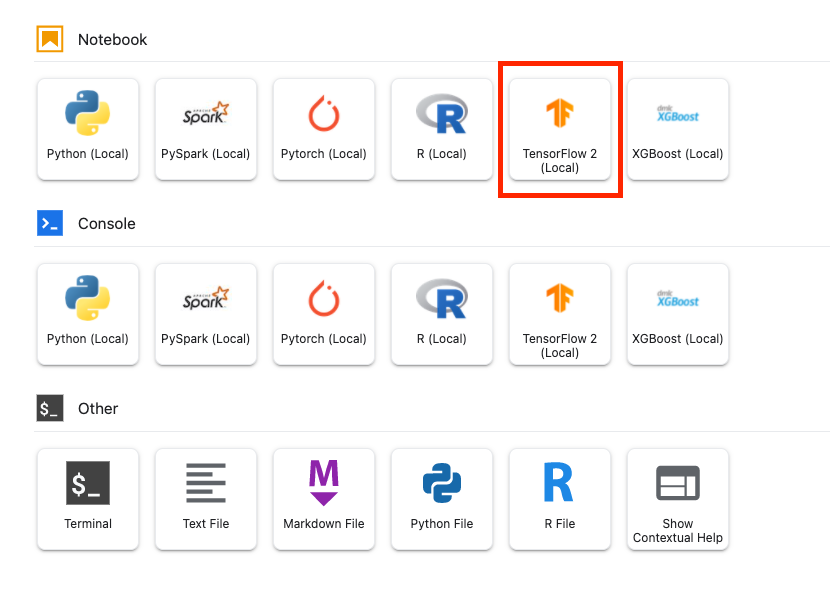``````from google.cloud import aiplatform
from google.cloud.aiplatform import hyperparameter_tuning as hpt
``````

``````# The spec of the worker pools including machine type and Docker image
# Be sure to replace PROJECT_ID in the `image_uri` with your project.

worker_pool_specs = [{
"machine_spec": {
"machine_type": "n1-standard-4",
"accelerator_type": "NVIDIA_TESLA_V100",
"accelerator_count": 1
},
"replica_count": 1,
"container_spec": {
"image_uri": "gcr.io/{PROJECT_ID}/horse-human:hypertune"
}
}]

# Dictionary representing metrics to optimize.
# The dictionary key is the metric_id, which is reported by your training job,
# And the dictionary value is the optimization goal of the metric.
metric_spec={'accuracy':'maximize'}

# Dictionary representing parameters to optimize.
# The dictionary key is the parameter_id, which is passed into your training
# job as a command line argument,
# And the dictionary value is the parameter specification of the metric.
parameter_spec = {
"learning_rate": hpt.DoubleParameterSpec(min=0.001, max=1, scale="log"),
"momentum": hpt.DoubleParameterSpec(min=0, max=1, scale="linear"),
"num_units": hpt.DiscreteParameterSpec(values=[64, 128, 512], scale=None)
}

``````

``````# Replace YOUR_BUCKET
my_custom_job = aiplatform.CustomJob(display_name='horses-humans-sdk-job',
worker_pool_specs=worker_pool_specs,
staging_bucket='gs://{YOUR_BUCKET}')
``````

``````hp_job = aiplatform.HyperparameterTuningJob(
display_name='horses-humans-sdk-job',
custom_job=my_custom_job,
metric_spec=metric_spec,
parameter_spec=parameter_spec,
max_trial_count=15,
parallel_trial_count=3)

hp_job.run()
``````

## 7. 清理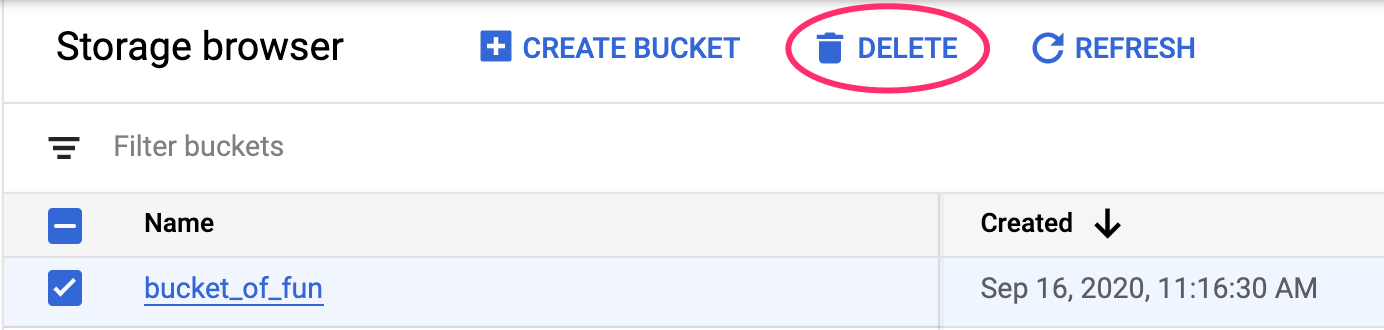[{ "type": "thumb-down", "id": "missingTheInformationINeed", "label":"没有我需要的信息" },{ "type": "thumb-down", "id": "tooComplicatedTooManySteps", "label":"太复杂/步骤太多" },{ "type": "thumb-down", "id": "outOfDate", "label":"内容需要更新" },{ "type": "thumb-down", "id": "translationIssue", "label":"翻译问题" },{ "type": "thumb-down", "id": "samplesCodeIssue", "label":"示例/代码问题" },{ "type": "thumb-down", "id": "otherDown", "label":"其他" }]
[{ "type": "thumb-up", "id": "easyToUnderstand", "label":"易于理解" },{ "type": "thumb-up", "id": "solvedMyProblem", "label":"解决了我的问题" },{ "type": "thumb-up", "id": "otherUp", "label":"其他" }]